# Introduction to Integrals and Integration by Transformation JEE Notes | EduRev

## Commerce : Introduction to Integrals and Integration by Transformation JEE Notes | EduRev

The document Introduction to Integrals and Integration by Transformation JEE Notes | EduRev is a part of the Commerce Course Mathematics (Maths) Class 12.
All you need of Commerce at this link: Commerce

A. INTRODUCTION
Both parts of the Fundamental Theorem establish connections between antiderivatives and definite integrals. Part 1 says that if f is continuous, then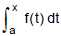is an antiderivative of f. Part 2 says that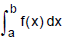can be found by evaluating F(b) – F(a), where F is an antiderivative of f. We need a convenient notation for antiderivative that makes them easy to work with. Because of the relation given by the Fundamental Theorem between antiderivative and integrals, the notation ∫f(x)dx is traditionally used for antiderivative of f and is called and indefinite integral.
Thus ∫ f(x)dx = F(x) + C

means F '(x) = f(x)

For example, we can write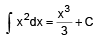because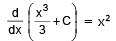So we can regard an indefinite integral as representing an entire family of function (one antiderivative for each value of the constant C).
You should distinguish carefully between definite and indefinite integrals. A definite integral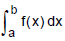is a number, whereas an indefinite integral ∫ f(x)dx is family of functions. The connection between them is given by part 2 of the Fundamental Theorem. If f is continuous on [a, b] then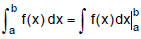The effectiveness of the Fundamental Theorem depends on having a supply of antiderivative of functions.

We adopt the convention that when a formula for a general indefinite integral is given, it is valid only on an interval. Thus, we write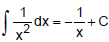With the understanding that it is valid on the interval (0, ∞) or on the interval (–∞, 0). This true despite the fact that the general antiderivative of the function f(x) = 1/x2, x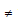0, is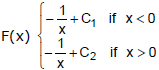ELEMENTARY INTEGRALS
(i) ∫ (ax + b)n dx = (ax + b)n+1/a(n+1) + c n ≠ - 1

(ii) ∫ dx/ax + b = 1/a ℓn |ax + b| + c

(iii) ∫ eax+b dx = 1/a eax+b + c

(iv) ∫ apx+1 dx = apx+q/pℓn a (a>0) + c

(v) ∫ sin (ax + b) dx = -1/a cos (ax + b) + c
(vi) ∫ cos (ax + b) dx = 1/a sin (ax + b) + c

(vii) ∫ cot (ax + b) dx = 1/a ℓn sin|ax + b| + c
(viii) ∫ tan (ax + b) dx =1/a ℓn sec |ax + b| + c
(ix) ∫ sec2 (ax + b) dx = 1/a tan (ax + b) + c
(x) ∫ cos ec2 (ax + b) dx = -1/a cot(ax + b) + c
(xi) ∫ sec (ax + b). tan (ax + b) dx = 1/a sec (ax + b) + c
(xii) ∫ sec x dx = In |sec x + tan x|
(xiii) ∫ cos ec x dx = In |cosec - cotx| + c

B. INTEGRATION BY TRANSFORMATION
Example 1. Integrate,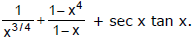Solution.
Here I = ∫ x-3/4 dx + ∫ (1 + x + x2 + x3)dx + ∫ sec x tan x dx
[∵ (1 - xn)/(1 - x) = 1 + x + x2 + x3 + ........ + xn-1]
∴ l = 4x1/4 + x + (x2/2) + (x3/3) + (x4/4) + sec x + c.

Example 2. Integrate (1 + sin x)/(1 – cos x).

Solution.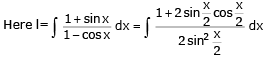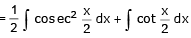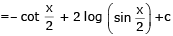Example 3. Evaluate,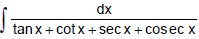Solution.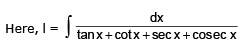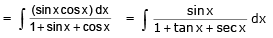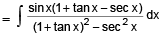Multiplying and dividing by (1 + tan x – sec x), we get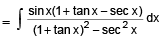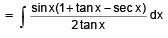= 1/2 ∫ cos x(1 + tan x - sec x) dx
= 1/2 ∫ (cos x + sin x - 1) dx = 1/2 ∫ (sin x - cos x - x) + c

Example 4. Integrate,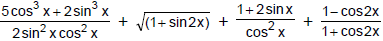Solution
The given expression may be written as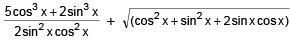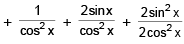= 5/2 cosec x cot x + sec x tan x + cos x + sin x + sec2 x + 2 sec x tan x + 2 (sec2 x – 1)
= 5/2 cosec x cot x + 3 sec x tan x + cos x + sin x + 3 sec2 x – 2.
Now integrating, we get
I = 5/2 ∫ cos ec cot x dx + 3 ∫ sec x tan x dx + ∫ cos x dx + ∫ sinx dx + 3 ∫ sec2 x dx - 2 ∫ dx
= - 5/2 cosec x + 3 sec x + sin x – cos x + 3 tan x – 2x + c.

Example 5. Integrate,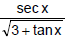Solution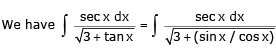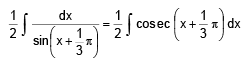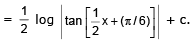CONSIDER INTEGRALS OF THE TYPES

∫ cos ax cos bx dx, ∫ cos ax cos bx dx, ∫ cos ax cos bx dx, in which a ≠ b.
We can use these addition formulae to change products to sums or differences, and the later can be integrated easily.

Example 6. Integrate  sin 8x sin 3x dx
Solution
sin 8x sin 3x = 1/2 (cos 5x - cos 11x),
and so,
∫ sin 8x sin 3x dx x = 1/2 ∫ (cos5x - cos11x) dx
= 1/10 sin 5x - 1/22 sin 11x + c
We next consider integrals of the type ∫ cosm x sinn x dx , in which at least one of the exponents m and n is an odd positive integer (the other exponent need only be a real number). Suppose that m = 2k + 1, where k is a non-negative integer. Then
cosmx sinnx = cos2k+1 x sinn x = (cos2x)k sinn x cos x.
Using the identity
cos2 x = 1 - sin2x, we obtain ∫ cosm .x sinn x dx = ∫ (1-sin2x)k sinn x cos x dx.
The factor (1 – sin2x)k can be expanded by the Binomial Theorem, and the result is that ∫ cosm x sinn x dx can be written as a sum of constant multiples of integrals of the form ∫ sinq x cos x dx.

Since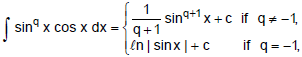If follows that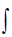cosm x sinn x dx can be readily evaluated, An entirely analogous argument follows if the exponent n is an odd positive integer.

Example 7. Integrate

(a) cos3 4x dx ,

(b) sin5 x cos4 x dx

Solution. In (a) illustrates that the method just described is applicable to odd positive integer powers of the sine or cosine (i.e., either m or n may be zero). We obtain
∫ cos3 4x dx = ∫ cos2 4x cos 4x dx = ∫ (1-sin2 4x)cos4x dx
= ∫ cos 4x dx - ∫ sin2 4x cos 4x dx  = 1/4 sin 4x - 1/12 sin34x + c.

ln (b) it is the exponent of the sine which is an odd positive integer.
∫ sin5 x cos4 x dx = ∫ (sin2x)2 cos4 x sin x dx
= ∫ (1 - cos2 x)2 cos4 x sin x dx
= ∫ (1 - 2cos2x + cos4x) cos4 x sin x dx
= ∫ cos4 x sin x dx - 2 ∫ cos6 x sin x dx + ∫ cos8 x sin x dx
= 1/5 cos5x + 2/7 cos7x - 1/9 cos9x + c.
The third type of integral we consider consists of those of the form ∫cosm x sinn x dx , in which both m and n are even non-negative integers. These function are not so simple to integrate as those containing an odd power. We first consider the special case in which either m = 0 or n = 0. Thesimplest non-trivial examples are the two integrals ∫ cos2 × dx and ∫ sin2 × dx, which can be integrated by means of the identities
cos2x = 1/2(1 + cos 2x), sin2x = 1/2 (1 - cos 2x)
Evaluation of the two integrals is now a simple matter. We get
∫ cos2 x dx = 1/2 ∫ (1 + cos2x) dx = x/2 + 1/4 sin 2x + c,
∫ sin2 x dx = 1/2 ∫ (1 - cos2x) dx = x/2 + 1/4 sin 2x + c.
Going on to the higher powers, consider the integral ∫ cos2i x dx, where i is an arbitrary positive integer.
We write,
cos2i x = (cos2 x)= [1/2(1+cos2x)]i = 1/2i(1 + cos 2x)i
We expand using binomial theorem and integrate the terms using previous methods.

Offer running on EduRev: Apply code STAYHOME200 to get INR 200 off on our premium plan EduRev Infinity!

## Mathematics (Maths) Class 12

209 videos|222 docs|124 tests

,

,

,

,

,

,

,

,

,

,

,

,

,

,

,

,

,

,

,

,

,

;